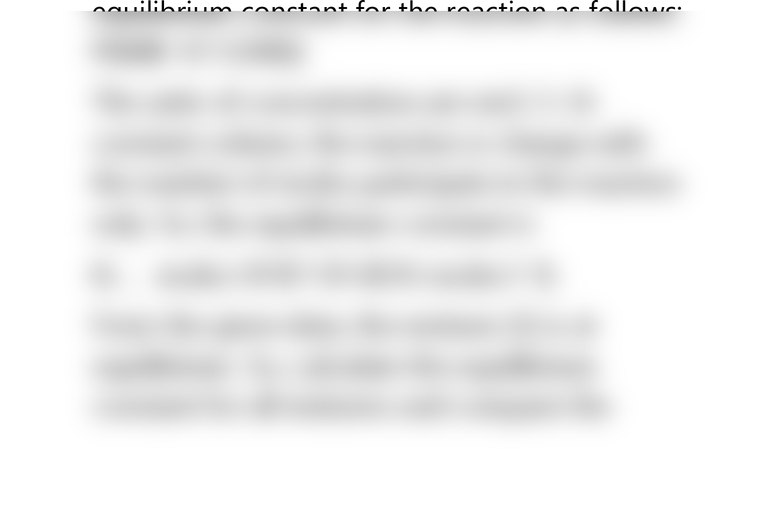# CAD-8104 Lecture Notes - Lecture 10: Equilibrium Constant

6 views2 pages
School
Department
Course
ProfessorCHEM 2426 HOMEWORK SOLUTIONS
The following pictures represent mixtures that
contain A atoms (red), B atoms (blue), and AB
and B, molecules, what interconvert accorchng
to the equation A w B, CAB + B. If mixture (1) is
at equilibrium, which of the other mixtures are
also at equilibrium? Explain.
The given reaction is A+B2 AB+B. Write the
equilibrium constant for the reaction as follows:
PkBilB K1 EARBi]
The units of concentration are mol / L At
constant volume, the reaction is change with
the number of moles participate in the reaction
only. So, the equilibrium constant is
Kc _ moles Of B7 Of AB fA moles f B,
From the given data, the mixture (1) is at
equilibrium. So, calculate the equilibrium
constant for all mixtures and compare the
Unlock document

This preview shows half of the first page of the document.
Unlock all 2 pages and 3 million more documents.